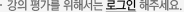## 주메뉴

### Numerical Simulations

• 경희대학교
• 테츠야 마가라• 주제분류
자연과학 >수학ㆍ물리ㆍ천문ㆍ지리 >우주과학
• 강의학기
2015년 2학기
• 조회수
2,741
•
Numerical simulation is a powerful and useful tool to understand complicated physical phenomena changing with time and space, where diffusion, convection, wave propagation and shock formation are involved. We start by learning basic features of partial differential equations (PDEs) that describe those phenomena. We then study how these equations are converted to the form suitable for numerical simulation. We also do a practical training of writing a numerical code and running a code to perform a simulation. Finally, we master how to use a data analysis software IDL (Interactive Data Language).

#### 차시별 강의1.강의계획서Introduction Numerical simulations of space plasmas IIntroduction Numerical simulations of space plasmas II2.Basic features of partial differential equations (PDEs) I Three types of PDEs IBasic features of partial differential equations (PDEs) I Three types of PDEs II3.Basic features of PDEs II Characteristics of PDEs IBasic features of PDEs II Characteristics of PDEs IIBasic features of PDEs II Characteristics of PDEs III4.Convert PDE to the form suitable for numerical simulation I Discretization of PDE5.Convert PDE to the form suitable for numerical simulation II Forward, centered and backward difference methods6.1-dimensional thermal conduction problem I Derive FTCS scheme for thermal conduction PDE7.1-dimensional thermal conduction problem II Explain basics of Fortran I1-dimensional thermal conduction problem II Explain basics of Fortran II8.Practical training of writing a code in computer languages How to write a code of FTCS scheme in FortranPractical training of writing a code in computer languages How to write data-analysis programs in IDL9.1-dimensional advection problem I Stability of numerical schemes I1-dimensional advection problem I Stability of numerical schemes II10.1-dimensional advection problem II FTCS scheme for advection PDE1-dimensional advection problem II Lax-Wendroff scheme for advection PDE11.Advanced numerical scheme for advection Numerical diffusion & oscillationAdvanced numerical scheme for advection Artificial viscosity in advection PDE12.Advanced numerical scheme for advection Flux limitor in advection PDE13.1-dimensional hydrodynamic problem I Fluid dynamic equations (FDEs) I1-dimensional hydrodynamic problem I FDEs II14.1-dimensional hydrodynamic problem II Conserved form of FDEs I1-dimensional hydrodynamic problem II Conserved form of FDEs II15.Advanced numerical scheme for hydrodynamics Upwind & Laxwendroff schemes for 1-dimensional FDEs and artificial viscosityAdvanced numerical scheme for hydrodynamics NormalizationShock wave problem Demonstrate numerical simulations of shock wave#### 연관 자료#### 사용자 의견